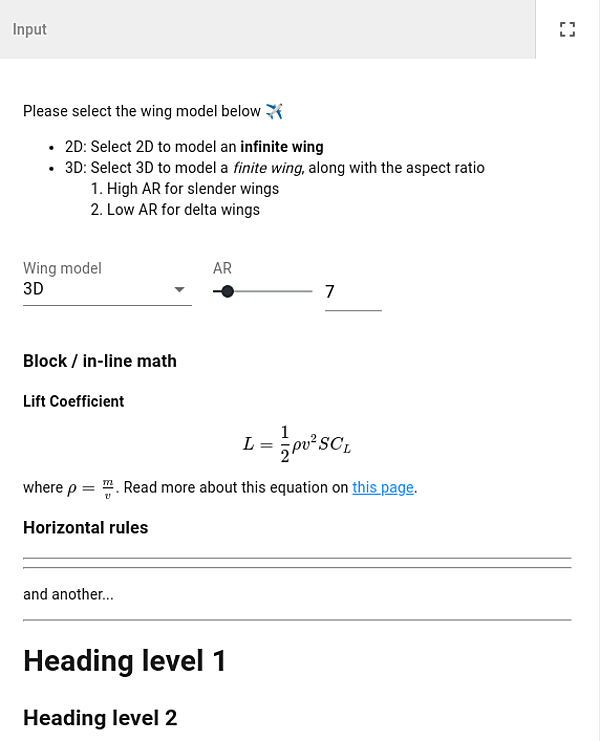# Text blocks

New in v12.7.0

A Text field that can be used to display a static text (max. 1800 characters) as follows:

class Parametrization(ViktorParametrization):    not_in_params = Text('My static text')
note

The value of the Text field is not included in the params.

The value of the Text fields can be styled using Markdown:from viktor.parametrization import ViktorParametrization, Text, OptionField, NumberFieldclass Parametrization(ViktorParametrization):    info1 = Text("""Please select the wing model below ✈️- 2D: Select 2D to model an **infinite wing**- 3D: Select 3D to model a *finite wing*, along with the aspect ratio    1. High AR for slender wings    2. Low AR for delta wings""")    model = OptionField("Wing model", options=["2D", "3D"])    ar = NumberField("AR", variant="slider", min=1, max=30)    info2 = Text("""### Block / in-line math#### Lift Coefficient$$L = \\frac{1}{2} \\rho v^2 S C_L$$where $\\rho = \\frac{m}{v}$. Read more about this equation on[this page](https://en.wikipedia.org/wiki/Lift_coefficient).### Horizontal rules------and another...---# Heading level 1## Heading level 2""")